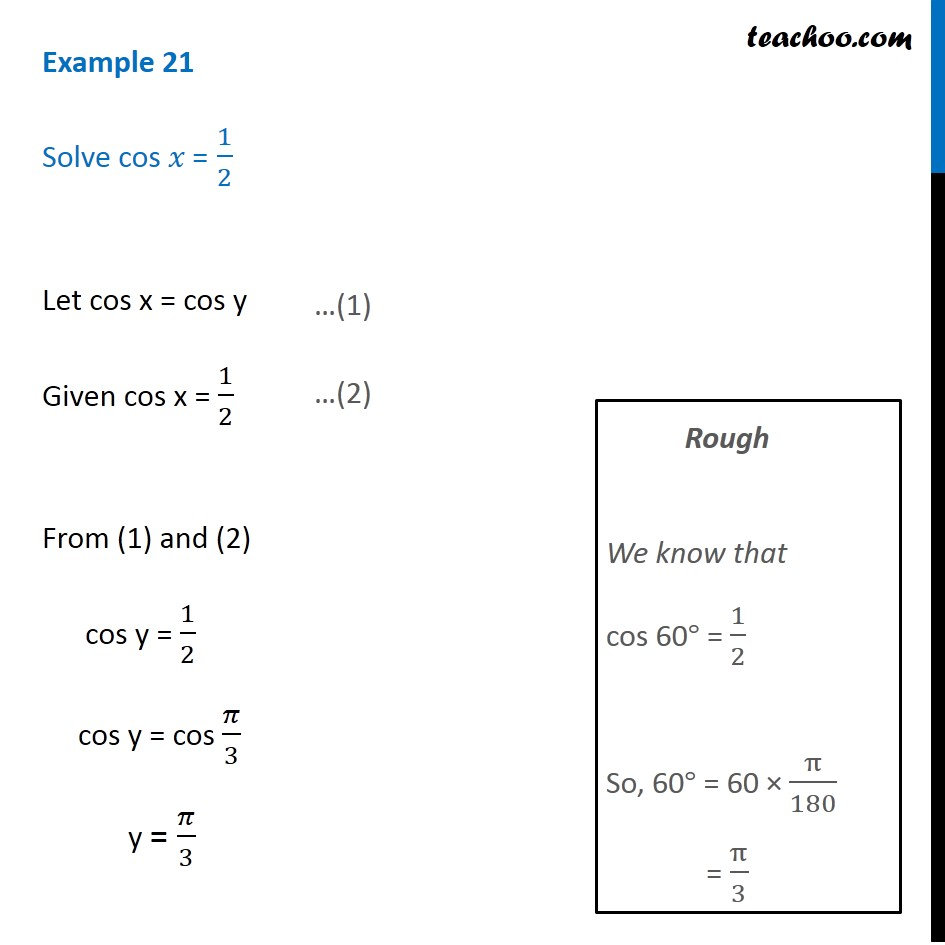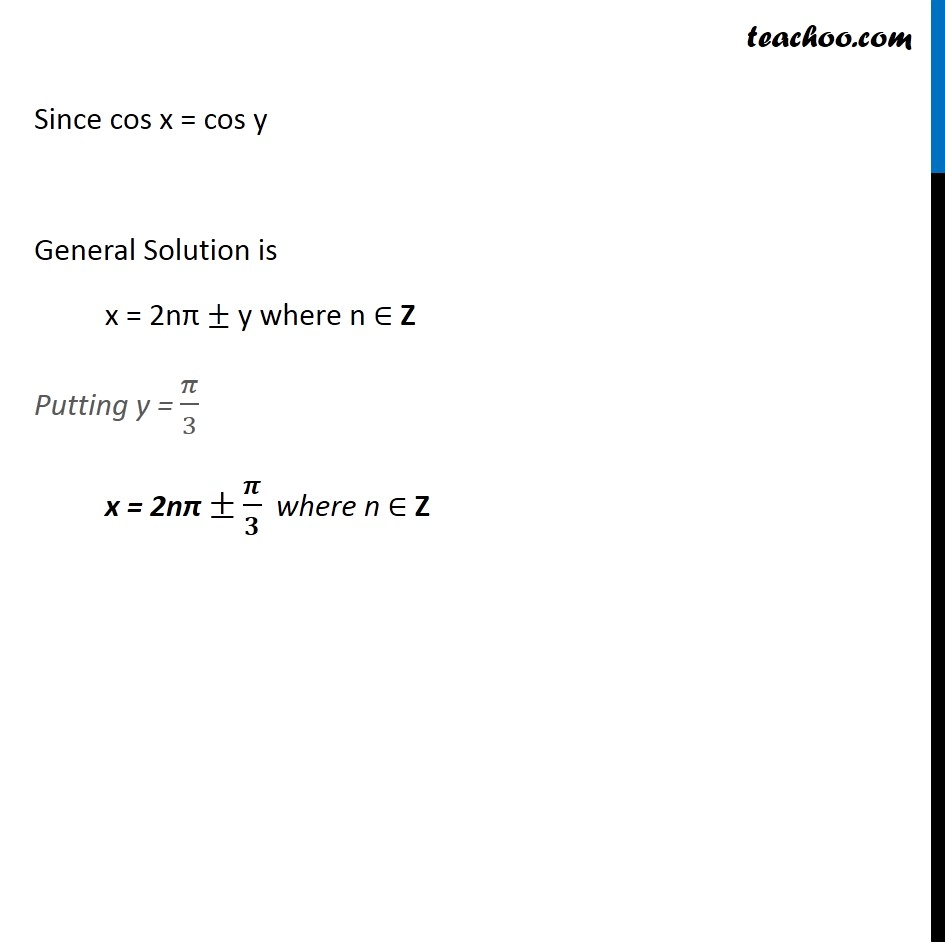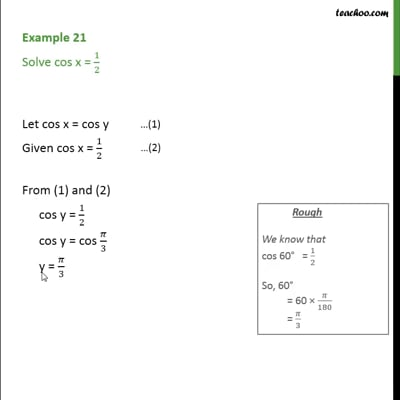Finding General Solutions

Chapter 3 Class 11 Trigonometric Functions
Concept wiseThis video is only available for Teachoo black users

Introducing your new favourite teacher - Teachoo Black, at only ₹83 per month

### Transcript

Example 21 Solve cos 𝑥 = 1/2 Let cos x = cos y Given cos x = 1/2 From (1) and (2) cos y = 1/2 cos y = cos 𝜋/3 y = 𝜋/3 Rough We know that cos 60° = 1/2 So, 60° = 60 × π/180 = π/3 Since cos x = cos y General Solution is x = 2nπ ± y where n ∈ Z Putting y = 𝜋/3 x = 2nπ ±𝝅/𝟑 where n ∈ Z